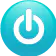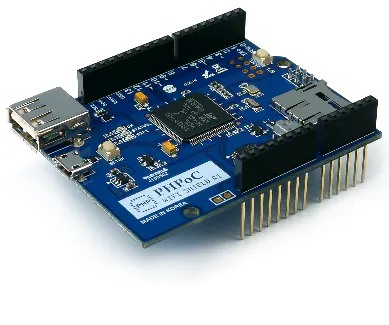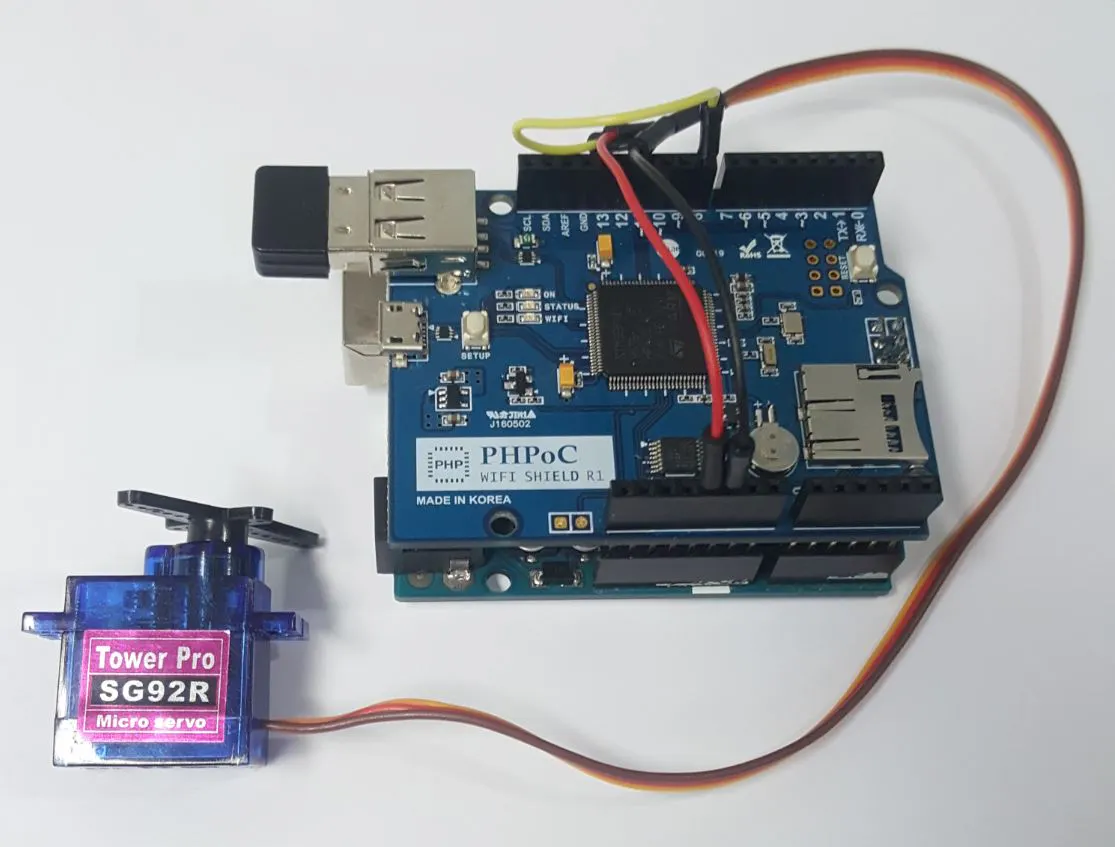# Arduino - Web Pattern Unlock

It's a security feature for Arduino. User is required to input the unlock pattern before remotely controlling/monitoring Arduino.

IntermediateFull instructions provided4 hours18,608

## Things used in this project

### Hardware componentsArduino UNO
×1PHPoC WiFi Shield for Arduino
×1
 Seeed Studio Servo Motor SG92R
×1
 Jumper wires
×1

## Schematics

### things_nuSqPThpdl.JPG## Code

### unlock.php

PHP
This is Web user interface
```<!DOCTYPE html>
<html>
<title>Arduino - PHPoC Shield</title>
<meta name="viewport" content="width=device-width, initial-scale=0.7, maximum-scale=0.7">
<meta charset="utf-8">
<style>
body { text-align: center; font-size: width/2pt; }
h1 { font-weight: bold; font-size: width/2pt; }
h2 { font-weight: bold; font-size: width/2pt; }
button { font-weight: bold; font-size: width/2pt; }
</style>
<script>

var CMD_AUTH = 0;
var CMD_CTRL = 1;
var ws;
var authorized = false;

/* unlock variable */
var unlock_width = 400, unlock_height = 400;
var unlock_gap = 140;
var unlock_touch_state = 0;
var unlock_touch_x = 0, unlock_touch_y = 0;
var unlock_touch_list = new Array();
var unlock_ratio = 1;

/* control variable: change as you want, as your application */
var servo_width = 401, servo_height = 466;
var servo_pivot_x = 200, servo_pivot_y = 200;
var servo_bracket_radius = 160, servo_bracket_angle = 0;
var servo_bracket_img = new Image();
var servo_click_state = 0;
var servo_last_angle = 0;
var servo_mouse_xyra = {x:0, y:0, r:0.0, a:0.0};

servo_bracket_img.src = "servo_bracket.png";

function init()
{
/* init unlock part */

var unlock = document.getElementById("unlock");
unlock.width = unlock_width;
unlock.height = unlock_height;

var ctx = unlock.getContext("2d");
ctx.translate(unlock_width/2, unlock_height/2);
ctx.lineCap="round";
ctx.lineJoin="round";

/* init control part */
var servo = document.getElementById("servo");

servo.width = servo_width;
servo.height = servo_height;
servo.style.backgroundImage = "url('/servo_body.png')";

ctx = servo.getContext("2d");
ctx.translate(servo_pivot_x, servo_pivot_y);

update_view();
}
function connect_onclick()
{
if(ws == null)
{
if((navigator.platform.indexOf("Win") != -1) && (ws_host_addr.charAt(0) == "["))
{
// network resource identifier to UNC path name conversion
}

ws = new WebSocket("ws://" + ws_host_addr + "/web_pattern", "text.phpoc");
document.getElementById("ws_state").innerHTML = "CONNECTING";
ws.onopen = ws_onopen;
ws.onclose = ws_onclose;
ws.onmessage = ws_onmessage;
}
else
ws.close();
}
function ws_onopen()
{
document.getElementById("ws_state").innerHTML = "<font color='blue'>CONNECTED</font>";
document.getElementById("bt_connect").innerHTML = "Disconnect";
update_view();
}
function ws_onclose()
{
document.getElementById("ws_state").innerHTML = "<font color='gray'>CLOSED</font>";
document.getElementById("bt_connect").innerHTML = "Connect";
ws.onopen = null;
ws.onclose = null;
ws.onmessage = null;
ws = null;
authorized = false;
update_view();
}
function ws_onmessage(e_msg)
{
e_msg = e_msg || window.event; // MessageEvent

var resp = parseInt(e_msg.data);

if(resp == 202)
authorized = true;
else if(resp == 401)
authorized = false;
else
console.log("unknown:" + resp);

update_view();
}
function update_view()
{
if(!authorized)
unlock_update_view();
else
servo_update_view();
}

function unlock_update_view()
{
document.body.style.backgroundColor = "black";
document.body.style.color = "white";

var unlock_area = document.getElementById('unlock_area');
var control_area = document.getElementById('control_area');

unlock_area.style.display = 'block';
control_area.style.display = 'none';

var unlock = document.getElementById("unlock");
var ctx = unlock.getContext("2d");

ctx.clearRect(-unlock_width/2, -unlock_height/2, unlock_width, unlock_height);

// draw touched point and line
ctx.lineWidth = 10;
ctx.strokeStyle="white";
ctx.globalAlpha=1;
ctx.beginPath();
for (var i = 0; i < unlock_touch_list.length; i++)
{
var temp = unlock_touch_list[i] - 1;
var x =  temp % 3 - 1;
var y = Math.floor(temp / 3) - 1;

ctx.lineTo(x*unlock_gap, y*unlock_gap);
}

if(unlock_touch_state)
ctx.lineTo(unlock_touch_x, unlock_touch_y);

ctx.stroke();

for (var i = 0; i < unlock_touch_list.length; i++)
{
var temp = unlock_touch_list[i] - 1;
var x =  temp % 3 - 1;
var y = Math.floor(temp / 3) - 1;

ctx.globalAlpha=0.2;
ctx.fillStyle = "white";
ctx.beginPath();
ctx.arc(x*unlock_gap, y*unlock_gap, unlock_outer_radius, 0, 2 * Math.PI);
ctx.fill();
}

// draw base
for(var y = -1; y <= 1; y++)
{
for(var x = -1; x <= 1; x++)
{
ctx.globalAlpha=0.5;
ctx.fillStyle = "white";
ctx.beginPath();
ctx.arc(x*unlock_gap, y*unlock_gap, unlock_middle_radius, 0, 2 * Math.PI);
ctx.fill();

ctx.globalAlpha=1;
ctx.fillStyle = "Cyan";
ctx.beginPath();
ctx.arc(x*unlock_gap, y*unlock_gap, unlock_inner_radius, 0, 2 * Math.PI);
ctx.fill();
}
}
}
function unlock_process_event(event)
{
if(event.offsetX)
{
unlock_touch_x = event.offsetX - unlock_width/2;
unlock_touch_y = event.offsetY - unlock_height/2;
}
else if(event.layerX)
{
unlock_touch_x = event.layerX - unlock_width/2;
unlock_touch_y = event.layerY - unlock_height/2;
}
else
{
unlock_touch_x = (Math.round(event.touches.pageX - event.touches.target.offsetLeft)) - unlock_width/2;
unlock_touch_y = (Math.round(event.touches.pageY - event.touches.target.offsetTop)) - unlock_height/2;
}

for(var i = 1; i <= 9; i++)
{
if(i == unlock_touch_list[unlock_touch_list.length - 1])
continue;

var idx_x = (i-1)%3 - 1;
var idx_y = Math.floor((i-1)/3) - 1;

var center_x = idx_x*unlock_gap;
var center_y = idx_y*unlock_gap;

var dist = Math.sqrt( (unlock_touch_x - center_x)*(unlock_touch_x - center_x) + (unlock_touch_y - center_y)*(unlock_touch_y - center_y) );

{
unlock_touch_list.push(i);
unlock_touch_state = 1;
break;
}
}

update_view();
}
function unlock_mouse_down()
{
if(ws == null || authorized)
return;

event.preventDefault();
unlock_process_event(event);
}
function unlock_mouse_up()
{
if(ws == null || authorized)
return;

event.preventDefault();

if(ws != null && authorized == false)
send_to_Arduino(CMD_AUTH, unlock_touch_list.toString());

unlock_touch_state = 0;
unlock_touch_list.splice(0, unlock_touch_list.length);
update_view();
}
function unlock_mouse_move()
{
if(ws == null || authorized)
return;

event.preventDefault();

if(authorized)
return;

unlock_process_event(event);
}

function servo_update_view()
{
document.body.style.backgroundColor = "white";
document.body.style.color = "black";

var unlock_area = document.getElementById('unlock_area');
var control_area = document.getElementById('control_area');

unlock_area.style.display = 'none';
control_area.style.display = 'block';

/* modify our control area here */
var servo = document.getElementById("servo");
var ctx = servo.getContext("2d");

ctx.clearRect(-servo_pivot_x, -servo_pivot_y, servo_width, servo_height);
ctx.rotate(servo_bracket_angle / 180 * Math.PI);

ctx.drawImage(servo_bracket_img, -servo_pivot_x, -servo_pivot_y);

ctx.rotate(-servo_bracket_angle / 180 * Math.PI);
}
function check_range_xyra(event, servo_mouse_xyra)
{
var x, y, r, a, rc_x, rc_y, radian;
var min_r, max_r, width;

if(event.touches)
{
var touches = event.touches;

x = (touches.pageX - touches.target.offsetLeft) - servo_pivot_x;
y = servo_pivot_y - (touches.pageY - touches.target.offsetTop);
min_r = 60;
max_r = servo_pivot_x;
width = 40;
}
else
{
x = event.offsetX - servo_pivot_x;
y = servo_pivot_y - event.offsetY;
min_r = 60;
width = 20;
}

/* cartesian to polar coordinate conversion */
r = Math.sqrt(x * x + y * y);
a = Math.atan2(y, x);

servo_mouse_xyra.x = x;
servo_mouse_xyra.y = y;
servo_mouse_xyra.r = r;
servo_mouse_xyra.a = a;

radian = servo_bracket_angle / 180 * Math.PI;

/* rotate coordinate */

if((r < min_r) || (r > max_r))
return false;

if((rc_y < -width) || (rc_y > width))
return false;

return true;
}
function servo_mouse_down()
{
if(event.touches && (event.touches.length > 1))
servo_click_state = event.touches.length;

if(servo_click_state > 1)
return;

if(check_range_xyra(event, servo_mouse_xyra))
{
servo_click_state = 1;
servo_last_angle = servo_mouse_xyra.a / Math.PI * 180.0;
}
}
function servo_mouse_up()
{
servo_click_state = 0;
}
function servo_mouse_move()
{
var angle;

if(event.touches && (event.touches.length > 1))
servo_click_state = event.touches.length;

if(servo_click_state > 1)
return;

if(!servo_click_state)
return;

if(!check_range_xyra(event, servo_mouse_xyra))
{
servo_click_state = 0;
return;
}

angle = servo_mouse_xyra.a / Math.PI * 180.0;

if((Math.abs(angle) > 90) && (angle * servo_last_angle < 0))
{
if(servo_last_angle > 0)
servo_last_angle = -180;
else
servo_last_angle = 180;
}

servo_bracket_angle += (servo_last_angle - angle);
servo_last_angle = angle;

if(servo_bracket_angle > 90)
servo_bracket_angle = 90;

if(servo_bracket_angle < -90)
servo_bracket_angle = -90;

servo_update_view();

send_to_Arduino(CMD_CTRL, Math.floor(servo_bracket_angle))

debug = document.getElementById("debug");
debug.innerHTML = Math.floor(servo_bracket_angle);

event.preventDefault();
}

function send_to_Arduino(cmd, data)
{
{
ws.send(cmd + ":" + data + "\r\n");
}
}

</script>

<body>

<p>
<h1>Arduino - Web Pattern Unlock</h1>
</p>
<div id="unlock_area" style="display:block;">
<canvas id="unlock"></canvas>
</div>
<div id="control_area" style="display:none;">
<canvas id="servo"></canvas>
<p>Angle : <span id="debug">0</span></p>
</div>
<h2>
<p>WebSocket : <span id="ws_state">null</span></p>
<button id="bt_connect" type="button" onclick="connect_onclick();">Connect</button>
</h2>

</body>
</html>
```

### ArduinoUnlockExample

Arduino
```/* arduino web server - pattern unlock */

#include "SPI.h"
#include "Phpoc.h"
#include <Servo.h>

#define CMD_AUTH    0
#define CMD_CTRL    1
#define ACCEPTED        "202"
#define UNAUTHORIZED    "401"

PhpocServer server(80);
Servo servo;
String pattern;
bool authenticated;
unsigned long timeout;
unsigned long lastActiveTime;

void setup() {
Serial.begin(9600);
while(!Serial)
;

Phpoc.begin(PF_LOG_SPI | PF_LOG_NET);
//Phpoc.begin();

server.beginWebSocket("web_pattern");

Serial.println(Phpoc.localIP());

servo.attach(8);  // attaches the servo on pin 8 to the servo object
servo.write(90);

pattern = String("1,4,8,6,3");
authenticated = false;
timeout = 10000; // 10000 milllisecond
lastActiveTime = 0;
}

void loop() {
// wait for a new client:
PhpocClient client = server.available();

if (client) {

if(data) {
int pos = data.indexOf(':');
int cmd = data.substring(0, pos).toInt();

if(cmd == CMD_AUTH) {
String reqPattern = data.substring(pos+1);

reqPattern.remove(reqPattern.indexOf(13));
reqPattern.remove(reqPattern.indexOf(10));

if(pattern.equals(reqPattern)) {
authenticated = true;
sendResponse(ACCEPTED, 3);
lastActiveTime = millis();
}
else {
//Serial.print(reqPattern);
authenticated = false;
sendResponse(UNAUTHORIZED, 3);
}
}
else
if(cmd == CMD_CTRL) {
if(authenticated) {
int angle = data.substring(pos+1).toInt();

//angle = map(angle, -90, 90, 0, 180);
angle = map(angle, 90, -90, 0, 180);

servo.write(angle);
lastActiveTime = millis();

Serial.println(angle);
}
else {
sendResponse(UNAUTHORIZED, 3);
}
}
}
}

if (authenticated && ((millis() - lastActiveTime) > timeout)){
authenticated = false;
sendResponse(UNAUTHORIZED, 3);
}
}

void sendResponse(char *data, int len) {
server.write(data, len);
}
```

## Credits

### phpoc_man

62 projects • 393 followers